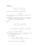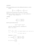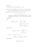MA158exam00pdfLoading previews...
 differential equations, first and second order Text Download (27kB)differential equations, first and second ordermatrices & determinants, eigenvalues and normalised eigenvectors, diagonal formlinear system of ODEs as a matrix problemdouble integrationtriple integration and sketching regions
6 files in this resource

MA158exam00pdf

Exam questions and solutions in PDFView Item